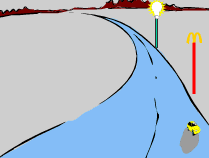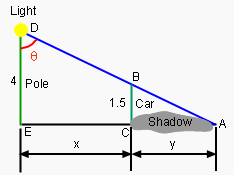Ch 2. Derivatives I Multimedia Engineering Math DerivativeFormulas TrigonometricFunctions Differentiability ChainRule ImplicitDifferentiation
 Chapter 1. Limits 2. Derivatives I 3. Derivatives II 4. Mean Value 5. Curve Sketching 6. Integrals 7. Inverse Functions 8. Integration Tech. 9. Integrate App. 10. Parametric Eqs. 11. Polar Coord. 12. Series Appendix Basic Math Units Search eBooks Dynamics Fluids Math Mechanics Statics Thermodynamics Author(s): Hengzhong Wen Chean Chin Ngo Meirong Huang Kurt Gramoll ©Kurt GramollMATHEMATICS - CASE STUDY IntroductionCar's shadow As Curtis drives from McDonalds at 5 m/sec, his daughter notes that as the distance between the car and the light increases, the shadow of the car gets longer. She knows that this is due to the changing angle made by the pole, the car and the light. She is curious about how fast this angle changes one second after starting to leave. What is known: The height of the pole is 4 m. The height of the car is 1.5 m. The speed of the car is 5 m/sec.The Relationship among the Pole, the Light and the Car Questions How fast does the angle that is made by the pole, the light and the car change 1 second after they start driving? Approach Derivative can be employed in this case.